Microsoft Excel Formula: How to Use TRIM function

TRIM function removes spaces from texts such spaces before or after the text and between texts.

Example:

WORD (Cell A2) and CONNECT (Cell B2) are combined as WORD CONNECT (Cell C2). However, there are spaces between those texts in Cell C2. To remove spaces, use the formula below.

=TRIM(cell where text spaces will be removed)
=TRIM(A2)   as seen in formula box when Cell D2 is clicked
=TRIM(B2)   as seen in formula box when Cell E2 is clicked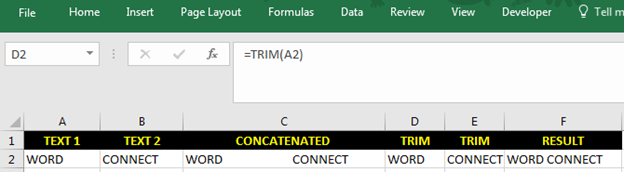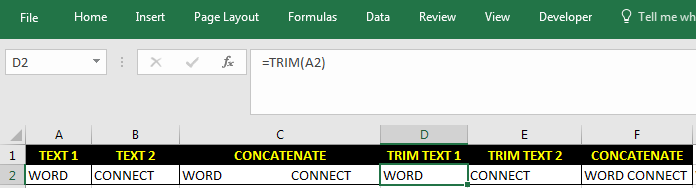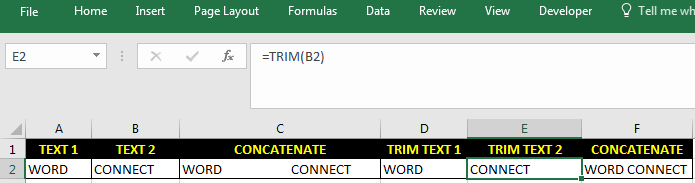To directly trim and combine the texts, use below.

=TRIM(A2)&" "&TRIM(B2))

or

=CONCAT(TRIM(A2)," ",B2)

See Cell F2 for result.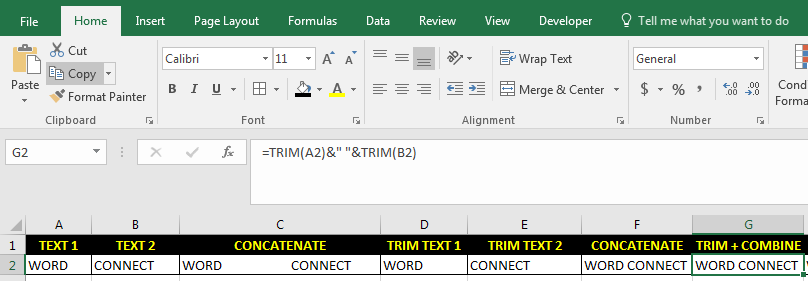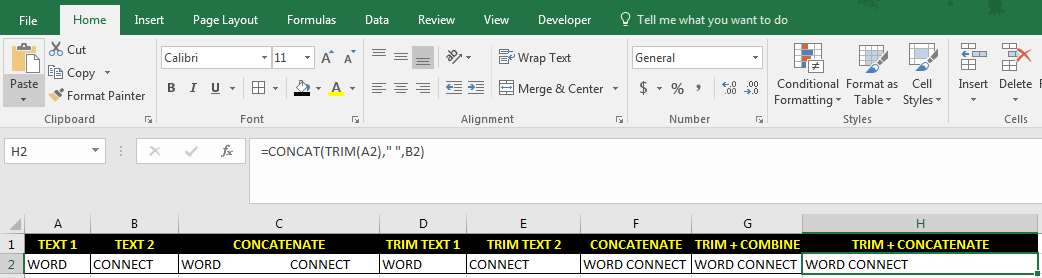Note: Excel version used is Microsoft Excel 2010